# International Workshop on Reliable Computing and Computer-Assisted Proofs (ReCAP 2022)

MAR.13 - 18, 2022

Maisaka: View of Lake-Hamana, "Fifty-three Stations on the Tokaido", No.31 by Hiroshige Ando

## New Information

• NEW February 21, 2022
We have decided ReCAP 2022 to be held fully online due to the recent spread of Covid-19.
• January 24, 2022
The deadline for onsite participation registration has been extended to February 24.
• January 11, 2022
The deadline for onsite participation registration has been extended.
• January 11, 2022
The deadline for submitting Title and Abstract has been extended.
• December 19, 2021
Website OPEN.
OVERVIEW

## Aim of ReCAP 2022

This workshop aims to strongly encourage mutual interaction among researchers in the fields of Reliable Computing and Computer-Assisted Proofs. Especially with the global expansion of Covid-19, many discussion forums have shrunk or gone online, reducing the number of interactive discussions with people outside of immediate research groups. As a result, it is more complex than ever to build trusting relationships with researchers with whom we have not previously interacted and form new communities. By using easy-to-use chat tools and the latest digital meeting devices, we can achieve a feeling similar to that of face-to-face meetings and at the same time achieve discussion efficiency higher than face-to-face meetings. The ReCAP workshop will bring back opportunities for interaction that were once commonplace.

## Important Dates

• Deadline for registration (ONSITE participation):   January 31 February 24, 2022 (Canceled)
• Deadline for submitting Title and Abstract:   January 31 February 15, 2022
• Deadline for registration (ONLINE participation):    February 28 March 12, 2022

ORGANIZERS

Kazuaki Tanaka (Waseda University)
Shin'ichi Oishi (Waseda University)
Takeshi Ogita (Tokyo Woman's Christian University)
Xuefeng Liu (Niigata University)
Akitoshi Takayasu (University of Tsukuba)

Student Staffs:
Taisei Asai (Waseda University)
Sora Sawai (Waseda University)

VENUE

All onsite events at Daiwa Royal Hotel "THE HAMANAKO" have been canceled, and ReCAP 2022 will be held fully online due to the recent spread of Covid-19.

REGISTRATION

Email:  recap2022(at)recap.sci.waseda.ac.jp

## TIME TABLE (IN JAPAN TIME)

Mar.13 (SUN) Discussion&Announcement

Program (PDF)

PROGRAM

## March 13 (Sunday)

Opening & Welcome Session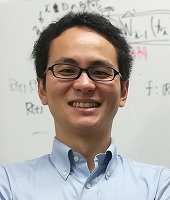Opening

Kazuaki Tanaka

Waseda University21:00--22:00

Welcome Session
Two Unsolved Problems in Computer Assisted Proofs of Nonlinear Delay Differential Equations

Shin'ichi Oishi

Waseda University

PROGRAM

## March 14 (Monday)

Plenary Talk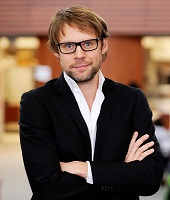21:00--22:00

The parameterization method: an overview, rigorous numerics and applications

Jean-Philippe Lessard

McGill University

ABSTRACT

In this talk, we review the fundamentals of the parameterization method, which provides a powerful way to obtain high order approximations of invariant manifolds in differential equations. The idea is first to introduce an invariance equation whose solution corresponds to the wanted parameterization, second to compute a numerical approximation of a solution of the invariance equation via power series and third to use the tools of rigorous numerics to validate the numerics. We present applications in the context of finite and infinite dimensional dynamical systems, namely ODEs, PDEs and delay equations.

Dynamical System 1Chebyshev interpolation for rigorous integrator of differential equations

Akitoshi Takayasu

University of Tsukuba

ABSTRACT

In this talk we consider the Chebyshev interpolation in particular for rigorous integrator of differential equations. Generally, to implement the Chebyshev interpolation, the number of Chebyshev polynomials that provides a sufficient approximation of the function depends on the original function to be approximated, and it is not obvious to determine the appropriate number of polynomials. We implement a heuristic algorithm to determine the appropriate number of polynomials and to interpolate the function with good accuracy. As an application, we introduce rigorous integration for the Cauchy problem of differential equations.Global dynamics and finite time blowup in a nonconservative nonlinear Schrödinger equation

Jonathan Jaquette

Boston University

ABSTRACT

In this talk we discuss the nonlinear Schrödinger equation iut = uxx + u2 posed on the circle. This NLS does not have gauge invariance and it does not admit a natural Hamiltonian structure.
In a recent series of papers, together with JP Lessard and A Takayasu, we have used computer assisted proofs to show that this equation exhibits rich dynamical behavior: such as non-trivial equilibria, homoclinic orbits, and heteroclinic orbits. Furthermore it turns out that the class of functions supported only on non-negative Fourier modes form an integrable subsystem, somewhat similar to the cubic Szegő equation. Within this class of initial data, we are able to explicitly construct solutions that are periodic, and solutions which blowup in finite time. This integrable subsystem is somewhat surprising, as it is also the case that the original PDE is nonconservative. I will conclude the talk with a discussion of some open problems.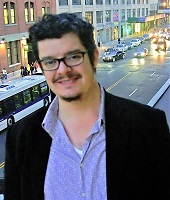Computer assisted proof of collision and near collision orbits in the circular restricted three body problem

Jason D. Mireles James

Florida Atlantic University

ABSTRACT

I will discuss some computer assisted existence proofs for ejection-collision orbits in the planar circular restricted three body problem. These are orbits which collide with of the primary bodies in forward time and collide with the other primary in backward time (the backward time collision is referred to as an ejection). Our argument exploits classical Levi-Civitta coordinates to regularize the singularities, and then formulates the collision orbits as the solution of a two-point boundary value problems in three different coordinate systems. We then employ a Newton-Kantorovich argument, and obtain existence, uniqueness, and transversality of the solution (transversality is relative to the energy level set). One technical point is that, to obtain a balanced system of equations in the energy manifold, we introduce an unfolding parameter which explicitly exploits the conserved quantities in the system. Essentially the same setup is used to compute other special orbits, like periodic orbits and homocliinics passing near or through collision. This is joint work with Shane Kepley and Maciej Capinski.Computing Morse decomposition of ODEs by non-rigorous numerics

Tomoyuki Miyaji

Kyoto University

ABSTRACT

In this talk we discuss methods of computing Morse decomposition for dynamical systems defined by ordinary differential equations. A sophisticated framework for computing Conley-Morse graphs has been proposed by Arai et al., [SIAM J. Appl. Dyn. Syst., 2009], which combines interval arithmetic, computational homology, and Conley indices. For ODEs, time-T maps or Poincare maps are solved by some rigorous integrator such as Lohner's method. However, it requires much computational costs. In order to reduce the costs, we resort to floating-point arithmetic for computing combinatorial multivalued maps. The proposed method uses numerical solution obtained by Runge-Kutta method and affine approximation of time-T maps or Poincare maps. We present numerical examples for time-T map of 3D ODE and Poincare map for 4D ODE.

EigenvaluesFully computable a posteriori error bounds for eigenfunctions

Tomas Vejchodsky

Institute of Mathematics, Czech Academy of Sciences

ABSTRACT

In the context of eigenvalue problems for compact self-adjoint operators in Hilbert spaces with multiple or tightly clustered eigenvalues, we present two fully computable a posteriori error bound for approximate eigenfunctions. The first one is straightforward to compute, but its accuracy is limited by the widths of the cluster and may deteriorate for higher parts of the spectrum. Therefore, we concentrate on the new and accurate bound motivated by Davis-Kahan's theorem, which overcomes these difficulties by estimating the dual norm of the residual by suitable flux reconstructions. The derived bounds apply well to linear elliptic operators discretized by the finite element method.Counterexamples in Spectral Geometry

Javier Gómez-Serrano

Brown University and University of Barcelona

ABSTRACT

In this talk I will explain how to construct counterexamples for two problems in spectral geometry. In the first part of the talk, I will explain how to prove that a triangle is not determined by its first, second and fourth (Dirichlet) eigenvalues, solving a conjecture by Antunes and Freitas. In the second part I will construct a planar domain with 6 holes for which the nodal line is closed and does not touch the boundary. In particular, this domain does not satisfy Payne’s nodal line conjecture. Both proofs are computer-assisted.
Joint work with Joel Dahne, Kimberly Hou and Gerard Orriols."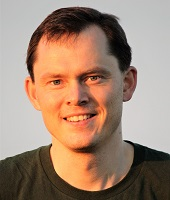Guaranteed bounds for eigenvalues and eigenvectors: multiplicities and clusters

Martin Vohralik

Inria Paris

ABSTRACT

We present a posteriori error estimates for conforming numerical approximations of eigenvalue clusters of second-order self-adjoint elliptic linear operators with compact resolvent. Given a cluster of eigenvalues, we estimate the error in the sum of the eigenvalues, as well as the error in the eigenvectors represented through the density matrix, i.e., the orthogonal projector on the associated eigenspace. This allows us to deal with degenerate (multiple) eigenvalues within the framework. All the bounds are valid under the only assumption that the cluster is separated from the surrounding smaller and larger eigenvalues; we show how this assumption can be numerically checked. Our bounds are guaranteed and converge with the same speed as the exact errors. They can be turned into fully computable bounds as soon as an estimate on the dual norm of the residual is available, which is presented in two particular cases: the Laplace eigenvalue problem discretized with conforming finite elements, and a Schrödinger operator with periodic boundary conditions discretized with planewaves. For these two cases, numerical illustrations are provided on a set of test problems.Rigorous eigenvalue estimation of the Stokes differential operators and computer-assisted proof of the solution to the Naiver-Stokes equation

Xuefeng LIU

Niigata University

ABSTRACT

In this talk, I will first introduce the problem of solution verification for the Navier-Stokes equation, where comes the need of rigorous eigenvalue estimation for differential operators. In the following part, a general framework for the eigenvalue estimation will be discussed. For the Stokes operator, both conforming and non-conforming finite element methods are utilized to obtain explicit eigenvalue bounds. Finally, there is a case report for the solution verification of the stationary Navier-Stokes equation over a 3D nonconvex domain.

PROGRAM

## March 15 (Tuesday)

Plenary Talk21:00--22:00

Accurate floating-point computations

Siegfried M. Rump

Hamburg University of Technology

ABSTRACT

Recently, although a very old subject, there was quite some progress in error estimates of floating-point operations. We discuss some details, starting with some tutorial intended for young researchers, trying in particular to give some encouragement for research. Following details of a new pair arithmetic are presented provably achieving accurate, i.e., faithfully rounded results for arbitrary arithmetic expressions.

Reliable Computing 1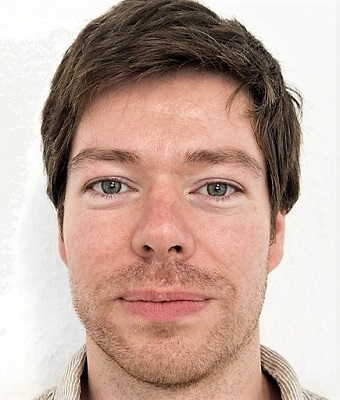An alternative approach to Ozaki's scheme for error-free transformation of matrix multiplication

Marko Lange

Hamburg University of Technology

ABSTRACT

The major advantage of Ozaki's scheme over several other approaches for computing accurate matrix-matrix products is the efficient use of highly optimized level-3 BLAS routines. Another benefit is the relatively low computational complexity for small boosts in precision. The biggest drawback is that the computational complexity and the required memory are increasing quadratically with the precision.
In this talk we discuss various improvements to Ozaki's original algorithm. We begin with a generalization of the theory for arbitrary bases of the underlying floating-point system and go on with modifying the actual splitting approach for a slightly increased accuracy. The focus of this talk lies on an alternative evaluation scheme to reduce the complexity of Ozaki's scheme.
We will further point out how the memory requirements of this scheme can be reduced drastically.Rayleigh quotient and accurate matrix multiplication for eigenvalue problems

Katsuhisa Ozaki

Shibaura Institute of Technology

ABSTRACT

In this talk, we introduce a method to obtain an accurate approximation of the eigenvalues of matrices using numerical computations. The target problems are the standard eigenvalue problem and the generalized eigenvalue problem. Approximate eigenvalues are obtained using the Rayleigh quotient with accurate matrix multiplication. Applying an error-free splitting of floating-point numbers, we propose a new method that uses mainly two matrix multiplications for the Rayleigh quotient for the standard eigenvalue problems. The proposed method can be applied to the generalized eigenvalue problems and singular value decomposition by the same argument. We also apply the proposed method to the iterative refinement for eigenvectors by Ogita and Aishima. Finally, we present numerical examples to illustrate the efficiency of the proposed method.Impact and Contribution of Ozaki scheme in High Performance Computing

Daichi Mukunoki

RIKEN Center for Computational Science

ABSTRACT

The Ozaki scheme, which was proposed as an accurate matrix multiplication method by Ozaki et al. in 2012, is not only useful for accurate computations, but also makes significant contributions to address issues in the field of high performance computing, such as reproducible computation and utilization of low-precision hardware. In this presentation, we will introduce some of our previous work on the Ozaki scheme, including its high-performance implementation, its application to reproducible computations, and its utilization of low-precision floating-point units on GPUs.

Differential Equations 1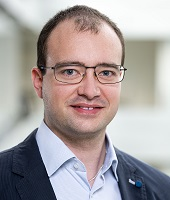Computer-assisted Existence Proofs for Navier-Stokes Equations on an Unbounded Strip with Obstacle I

Jonathan Wunderlich

Karlsruhe Institute for Technology (KIT)

ABSTRACT

The incompressible stationary 2D Navier-Stokes equations are considered on an unbounded strip domain with a compact obstacle, and with the Poiseuille flow as a background flow near infinity. First, a computer-assisted existence and enclosure result for the velocity (in a suitable divergence-free Sobolev space) is presented. Starting from an approximate solution (computed with divergence-free finite elements), we determine a bound for its defect, and use a norm bound for the inverse of the linearization at the approximate solution. The computation of such a norm bound will be described in the subsequent talk. Finally, if the computer-assisted proof provides the existence of a velocity field, the existence of a corresponding pressure can be obtained by purely analytical techniques. Nevertheless, for a given approximate solution to the pressure our methods provide an error bound (in a dual norm) as well.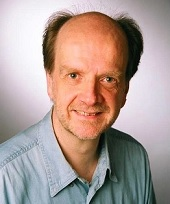Computer-assisted Existence Proofs for Navier-Stokes Equations on an Unbounded Strip with Obstacle II

Michael Plum

Karlsruhe Institute of Technology

ABSTRACT

The most challenging issue in computer-assisted proofs for nonlinear boundary value problems is the computation of a norm bound for the inverse of the linearization at a numerical approximate solution to the nonlinear problem. Several approaches to this task are known, but for unbounded domains the only available option appears to be via eigenvalue bounds, supplemented by bounds to the essential spectrum. We illustrate this method by applying it to the Navier-Stokes problem on an unbounded strip domain with obstacle considered in the previous talk. We use the Rayleigh-Ritz method, the Temple-Lehmann-Goerisch method, and a homotopy method for obtaining the needed spectral pre-information. In detail, three homotopies are performed; the first deforms the coefficients into piecewise constant ones, the second deforms the domain with obstacle into the full strip, and the third fades out the divergence condition.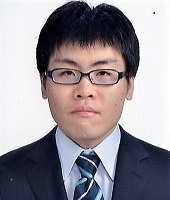Numerical verification method for a blow-up solution of Fujita-type equation

Makoto Mizuguchi

Chuo University

ABSTRACT

In this talk, we propose a rigorous numerical verification method obtaining an enclosure of a blow-up time for the initial-Dirichlet boundary value problems for Fujita-type equation. The lower bound of the blow-up time is given by a computer-assisted proof of a solution for parabolic equations. The results by the computer-assisted proof and a fundamental theory of ordinary differential inequality using the Energy functional not only show that a solution blows up in finite time but also provide an upper bound of the blow-up time. The enclosure of the blow-up time can be obtained by the lower and upper bounds. We show the effectiveness of our method throughout numerical results.

PROGRAM

## March 16 (Wednesday)

Plenary Talk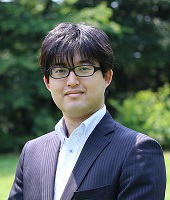21:00--22:00

Summary of Numerical Verification Methods for Solutions to infinite dimensional problems on Banach spaces using a projection

Kouta Sekine

Chiba institute of technology

ABSTRACT

In this talk, we introduce numerical verification methods of infinite dimensional problems on Banach spaces using projection. We will start with an introduction to Kantorovich's theorem and introduce the transition from the Newton-like method to the exact Newton method. In particular, the proposed method of infinite dimensional Gaussians elimination with Schur complement also be presented.

Differential Equations 2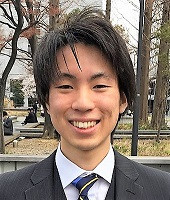Computer-assisted analysis for the bifurcation phenomena of the one-dimensional Hénon-type equation

Taisei Asai

Waseda University

ABSTRACT

In this talk, we present the numerical verification method for the one-dimensional Hénon-type equation. The Hénon equation is well known as an equation that causes the symmetry breaking bifurcations, but recently, it is expected that the Hénon-type equation will cause more diverse bifurcations. Therefore, we tracked the bifurcation diagrams of the equation with numerical verification. As a result, we found up to 7 multiple solutions at a certain parameter value and proved their existence.A computer-assisted proof toward the critical Reynolds number for the Orr-Sommerfeld problem

Yoshitaka Watanabe

Kyushu University

ABSTRACT

The present talk describes a computer-assisted proofs for the Orr-Sommerfeld equation describing the hydrodynamic stability of Poiseuille flow. Using spectral Galerkin approximate solutions bounding its small defect and a fixed-point theorem, an enclosure of a strong candidate of the critical Reynolds number is shown.On some convergence properties of approximate inverse operator norm in FEM

Mitsuhiro Nakao

Waseda University

ABSTRACT

In this talk, we present some convergence theorems of the Galerkin finite element approximation for the second order elliptic boundary value problems. Under the quite general setting, we show not only the pointwise convergence but also prove that the norm of approximate operator converges to the corresponding norm for the inverse of linear elliptic operator. Since the approximate norm estimates of linearized inverse operator plays an essential role in the numerical verification method of solutions for nonlinear elliptic problems, our result is also important in terms of guaranteeing its validity. Furthermore, the present method can also be applied to more general elliptic problems, e.g., biharmonic problems and so on.

Reliable Computing 2On a compensated Ehrlich-Aberth method for the accurate computation of all polynomial roots

Stef Graillat

Sorbonne University

ABSTRACT

In this talk, we will use the complex compensated Horner’s method to derive a compensated Ehrlich-Aberth method for the accurate computation of all roots of a polynomial. In particular, under suitable conditions, we will show that the limiting accuracy for the compensated Ehrlich-Aberth iterations is as accurate as if computed in twice the working precision and then rounded into the working precision. Moreover, we will present a running error bound for the complex compensated Horner’s and use it to form robust stopping criteria for the compensated Ehrlich-Aberth iterations. Finally, extensive numerical experiments will illustrate that the backward and forward errors of the root approximations computed via the compensated Ehrlich-Aberth method are similar to those obtained with a quadruple precision implementation of the Ehrlich-Aberth method with a significant speed-up in terms of the computation time. This is a joint work with Thomas Cameron.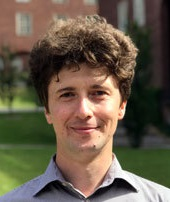General approach for re-assuring reproducibility of iterative solvers

Roman Iakymchuk

Sorbonne University / Umea University

ABSTRACT

Parallel implementations of Krylov subspace algorithms often help to accelerate the procedure to find the solution of a linear system. However, from the other side, such parallelization coupled with asynchronous and out-of-order execution often enlarge the non-associativity of floating-point operations. This results in non-reproducibility on the same or different settings. This paper proposes a general framework for deriving reproducible and accurate variants of a Krylov subspace algorithm. The proposed algorithmic strategies are reinforced by programmability suggestions to assure deterministic and accurate executions. The framework is illustrated on the preconditioned BiCGStab method for the solution of non-symmetric linear systems in parallel environments with message-passing. Finally, we verify the two reproducible variants of PBiCGStab on a set matrices from the SuiteSparse Matrix Collection.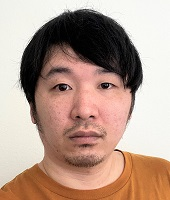Efficient GMRES-based Iterative Refinement for Ill-conditioned Matrices

Takeshi Terao

RIKEN Center for Computational Science

ABSTRACT

We discuss a GMRES-based iterative refinement for ill-conditioned matrices. It is known that the GMRES-based iterative refinement (GMRES-IR) proposed by Carson and Higham is more robust than the conventional iterative refinement. The GMRES-IR method applies a preconditioned GMRES method using computed LU factors to correction equations. In this study, we modify the preconditioning in GMRES-IR in terms of computational cost and stability. When an LU decomposition is performed in lower precision for ill-conditioned matrices, it is likely that computed LU factors contain unnecessary information. By avoiding computation of unnecessary elements, we can reduce the computational cost of LU decomposition. In addition, we can also reduce the computational cost of forward and backward substitutions, which is dominant in GMRES with preprocessing. We will present numerical results showing performance of our proposed method.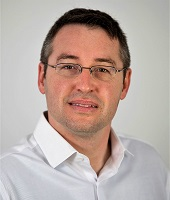Iterative Refinement of Schur decompositions

Daniel Kressner

EPFL, Swiss

ABSTRACT

The Schur decomposition of a square matrix A is an important intermediate step of state-of-the-art numerical algorithms for addressing eigenvalue problems, matrix functions, and matrix equations. This talk is concerned with the following task: Compute a (more) accurate Schur decomposition of A from a given approximate Schur decomposition. This task arises, for example, in the context of parameter-dependent eigenvalue problems and mixed precision computations. We have developed a Newton-like algorithm that requires the solution of a triangular matrix equation and an approximate orthogonalization step in every iteration. We prove local quadratic convergence for matrices with mutually distinct eigenvalues and observe fast convergence in practice. In a mixed low-high precision environment, our algorithm requires only four high-precision matrix-matrix multiplications per iteration. When refining double to quadruple precision, it often needs only 3-4 iterations, which reduces the time of computing a quadruple precision Schur decomposition by up to a factor of 10-20.

PROGRAM

## March 17 (Thursday)

Plenary Talk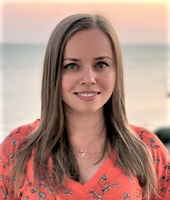21:00--22:00

Towards automatic and reliable roundoff error analysis for Deep Neural Networks

Anastasia Volkova

University of Nantes

ABSTRACT

Deep Neural Networks (DNN) represent a performance-hungry application. Floating-Point (FP) and custom FP-like arithmetic satisfies this hunger. While there is need for speed, inference in DNNs does not seem to have any need for precision. Many papers experimentally observe that DNNs can successfully run at almost ridiculously low precision.
The aim of this talk is two-fold: first, to shed some theoretical light upon why a DNN's FP accuracy stays high for low FP precision. We observe that the loss of relative accuracy in the convolutional steps is recovered by the activation layers, which are extremely well-conditioned. We give an interpretation for the link between precision and accuracy in DNNs.
Second, we present a software framework for automated FP error analysis for the DNN inference. Compatible with common Tensorflow/Keras models, it transforms a neural network into C++ code in order to analyze the network's need for precision. This rigorous analysis is based on Interval and Affine arithmetics to compute absolute and relative error bounds for a DNN.

Neural NetworkRigorous solution-enclosures of differential equations between sub- and super-solutions constructed by neural networks

Kazuaki Tanaka

Waseda University

ABSTRACT

We propose a method for enclosing a solution of differential equations between neural networks. The method is based on a sub- and super-solutions argument and mainly explained with an application to initial values problems of ODEs but can be extended to elliptic and parabolic PDEs. We will show examples where sub- and super solutions are constructed by neural networks, and the solution existence is proved between them.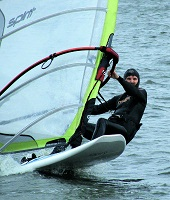Towards interval based verification of Artificial neural networks

Tibor Csendes

University of Szeged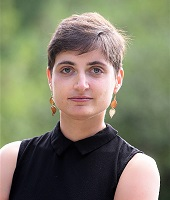Computer Validation of Neural Networks, a first case study

Elena Queirolo

Technische Universität München

ABSTRACT

By viewing recurrent neural networks as dynamical systems, a variety of mathematical tools becomes readily applicable. We are here interested in giving an initial result on the use of validated numerics to understand the dynamical behaviour of neural networks. As a case study, we consider a class of recurrent neural networks, where we prove via computer assistance the existence of several hundred Hopf bifurcation points, their non-degeneracy, and hence also the existence of several hundred periodic orbits. Our paradigm has the capability to rigorously verify complex nonlinear behaviour of neural networks, which provides a first step to explain the full abilities, as well as potential sensitivities, of machine learning methods via computer-assisted proofs.

Dynamical System 2On verification methods for conservative systems

Nobito Yamamoto

The University of Electro-Communications

ABSTRACT

We treat verification methods for ODEs with conserved quantity based on Lohner method (AWA). Using the information obtained from the calculation of the conserved quantity, we show a new aspect of verification of the solutions, especially on time intervals.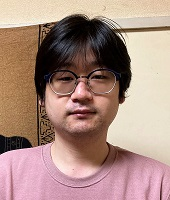On numerical verification methods to construct local Lyapunov functions around non-hyperbolic equilibria for two-dimensional cases

Koki Nitta

The University of Electro-Communications

ABSTRACT

In this talk, we propose numerical verification methods to construct local Lyapunov functions around non-hyperbolic equilibria. Our method uses nonlinear transformations inspired by the normal form theory of dynamical systems. We indicate the sufficient conditions to construct local Lyapunov functions and the method to construct them. Furthermore, we show an example problem where we have to use a fifth-degree polynomial to construct local Lyapunov functions.Computer assisted proofs for spiral waves in the complex Ginzburg-Landau problem

Jan Bouwe van den Berg

Vrije Universiteit Amsterdam

ABSTRACT

The cubic complex Ginzburg-Landau equation is among the prototypical systems in the study of pattern formation. One of its dynamic features is the occurence of rotating spirals. Due to special symmetries of this partial differential equation, a spiral wave Ansatz reduces the problem to a nonautonomous ordinary differential equation. Finding spiral waves thus corresponds to establishing certain connecting orbits in a finite dimensional dynamical system. In this talk we discuss how to approach this problem via computer assisted proof techniques. We use a domain decomposition which allows a combination of Taylor series, Chebyshev series and the parametrization of a center-stable manifold.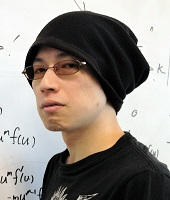Recent progress in blow-up characterization of ODEs: theory and rigorous numerics

Kaname Matsue

Kyushu University

ABSTRACT

Recent progress in studies for blow-up solutions of ODEs are summarized. Standard theory of dynamical systems and asymptotic analysis suitable for rigorous numerics are well applied to blow-up characterization of ODEs. In this talk, qualitative and quantitative features of blow-up solutions via parameterization method are mainly shown. If time permits, a perspective through asymptotic analysis is also addressed.

CONTACT